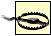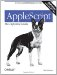# Section 15.2. Arithmetic Operators

### 15.2. Arithmetic Operators

The arithmetic operators combine numbers to get new numbers in accordance with the usual rules of arithmetic. As in most computer languages, multiplication and division take precedence over addition and subtraction (in the absence of parentheses). So, for example:

` 3 + 4 * 2 -- 11 3 * 4 + 2 -- 14 `

An operand that is a list consisting of one number will be coerced to a number. An operand that is a string representing a number, or a list consisting of one such string, will be coerced to a number.

The class of the result of the addition, subtraction, multiplication, and remainder operators is as follows: the result is an integer if the first operand is an integer and if the second operand either is an integer or is a real that can be coerced to an integer without loss of information. Otherwise, the result is a real.If you have some programming experience and you know a language such as C or LISP (where if either arithmetic operand is a real, the result is a real), you're going to find AppleScript's behavior surprising. Think of it like this. For the result to be a real, it is not enough that one operand be a real; the first operand must be a real, or else the second operand must have a significant fractional part, not merely a decimal point. Saying x * 1.0, for example, is not a way of making sure you've got a real. When in doubt, coerce explicitly.

Do not blame AppleScript for the phenomena inherent in doing floating-point arithmetic in any language on any computer. It is the nature of computer numerics that most values can only be approximated. Modern processors are extraordinarily clever about compensating, but rounding operations can easily expose the truth:

` 2.32 * 100.0 div 1 -- 231 `

Similarly, there may be situations where instead of comparing two values for absolute equality, you will do better to test whether the difference between them lies within some acceptable small epsilon.

 +

addition

#### Syntax

` number1 + number2 date + integer`

#### Description

Adds the operands. The addition operator is not overloaded to perform string concatenation; see "Concatenation Operator" (&) later in this chapter. On date arithmetic, see Chapter 13.

 -

subtraction ; unary negation

#### Syntax

` number1 - number2 date - integer date - date-number `

#### Description

Subtracts the second operand from the first, or negates the single operand. Unary negation has very high precedence . On date arithmetic, see Chapter 13.

 *

multiplication

#### Syntax

` number * number `

#### Description

Multiplies the operands.

 /

real division

#### Syntax

` number1 / number2 `

#### Description

Divides the first operand by the second. Both numbers are treated as reals, and the result is a real.

 div

integer division

#### Syntax

` number1 div number2 `

#### Description

Obtains the integer part of a division. Both numbers are treated as reals; the first is divided by the second, and the result is coerced to an integer by throwing away its fractional part. Notice that this is not the same as AppleScript's normal real-to-integer coercion behavior. Thus, the way to get the integer part of a real in AppleScript is x div 1, not x as integer.

#### Example

` 4 div 5 -- 0 (4 / 5) as integer -- 1 `

 mod

remainder

#### Syntax

` number1 mod number1 `

#### Description

Obtains the remainder from a division. The first operand is divided by the absolute value of the second, and the remainder is returned.

 ^

exponentiation

#### Syntax

` number1 ^ number2 `

#### Description

Raises the first number to the power of the second. The result is a real.AppleScript: The Definitive Guide, 2nd Edition
ISBN: 0596102119
EAN: 2147483647
Year: 2006
Pages: 267
Authors: Matt Neuburg

Similar book on Amazon

flylib.com © 2008-2017.
If you may any questions please contact us: flylib@qtcs.net